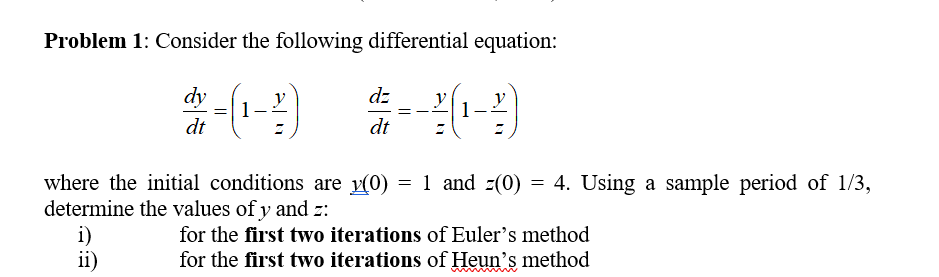# Problem 1: Consider the following differential equation: dy dt where the initial conditions are (0)1 and (0) 4. Using a sample period of 1/3, determine the values of y and : for the first two iterations of Euler's method for the first two iterations of Heun's method

Questionhelp_outlineImage TranscriptioncloseProblem 1: Consider the following differential equation: dy dt where the initial conditions are (0)1 and (0) 4. Using a sample period of 1/3, determine the values of y and : for the first two iterations of Euler's method for the first two iterations of Heun's method fullscreen

### Want to see the step-by-step answer?